# Exterior and interior boundary value problems

(diff) ← Older revision | Latest revision (diff) | Newer revision → (diff)

Boundary value problems for elliptic partial differential equations in the finite (interior) domain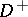, respectively in the infinite (exterior) domain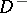into which a given closed smooth surface, homeomorphic to a sphere, subdivides the Euclidean space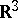.

The principal difference between exterior and interior boundary value problems consists in the fact that in the former case the boundary condition must be supplemented by a stipulation as to a certain behaviour at infinity, which ensures that the solution is unique and which is natural from the point of view of the physical origin of the problem itself.

For instance, in the exterior boundary value problems for the Poisson equation(the functionis assumed to be sufficiently smooth and of compact support) it is sufficient to stipulate that the solution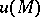be regular at infinity, i.e. that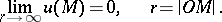(1)

In the case of the Poisson equationin an infinite domain in the plane,, the regularity condition at infinity is reduced to stipulating that the solutionbe bounded at infinity: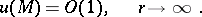(2)

In the case of the exterior boundary value problem for the Helmholtz equation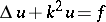,, the stipulation of regularity at infinity proves to be insufficient to obtain a unique solution. Here the so-called radiation conditions are employed. For the domainin: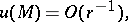(3)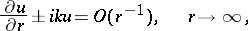and for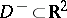:(4)the signs being chosen depending on the conditions of the problem and the choice of a principal fundamental solution. About other conditions at infinity see Limit-absorption principle; Limiting-amplitude principle.

Consider a boundary value problem for a linear elliptic equation of general form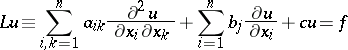(5)

in the domainsandof the Euclidean space,, separated by a closed smooth hypersurfacethat is homeomorphic to the sphere in, the functions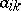,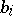,, andbeing assumed sufficiently smooth, andbeing of compact support. The conditions of regularity at infinity of type (1) or (2) will be sufficient in exterior boundary value problems ifor, if the maximum principle is satisfied by the operatorand if there exists a single unique principal fundamental solution; in particular, a necessary condition for it is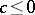(see , , ). The problem of the applicability of the radiation conditions, the principle of limit absorption and the limiting-amplitude principle in their general form has not yet (1977) been exhaustively investigated.

In addition to the difference between the conditions at infinity, an exterior and interior boundary value problem may also differ by the conditions for existence of the solution. Thus, in the case of the interior Neumann problem for the Laplace equation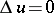in a finite domain, a necessary condition for the existence of a solution has the formwhereis the given boundary function in the Neumann condition. However, this condition is no longer necessary in the exterior Neumann problem in an infinite domain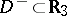.

How to Cite This Entry:
Exterior and interior boundary value problems. Encyclopedia of Mathematics. URL: http://encyclopediaofmath.org/index.php?title=Exterior_and_interior_boundary_value_problems&oldid=13211
This article was adapted from an original article by E.D. Solomentsev (originator), which appeared in Encyclopedia of Mathematics - ISBN 1402006098. See original article